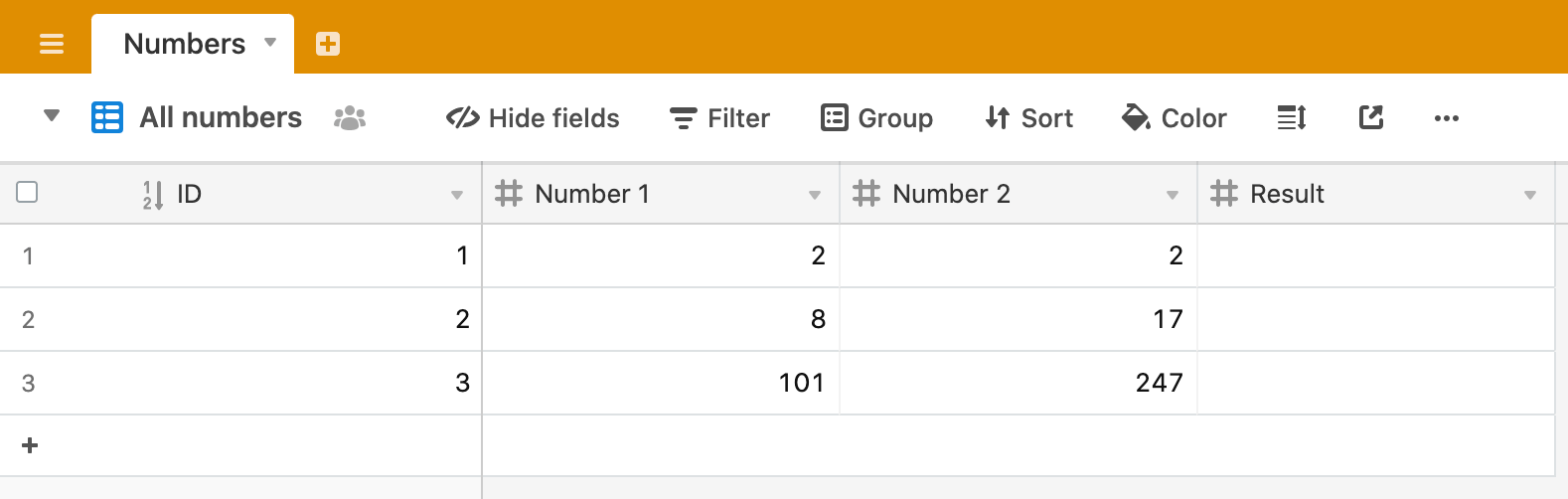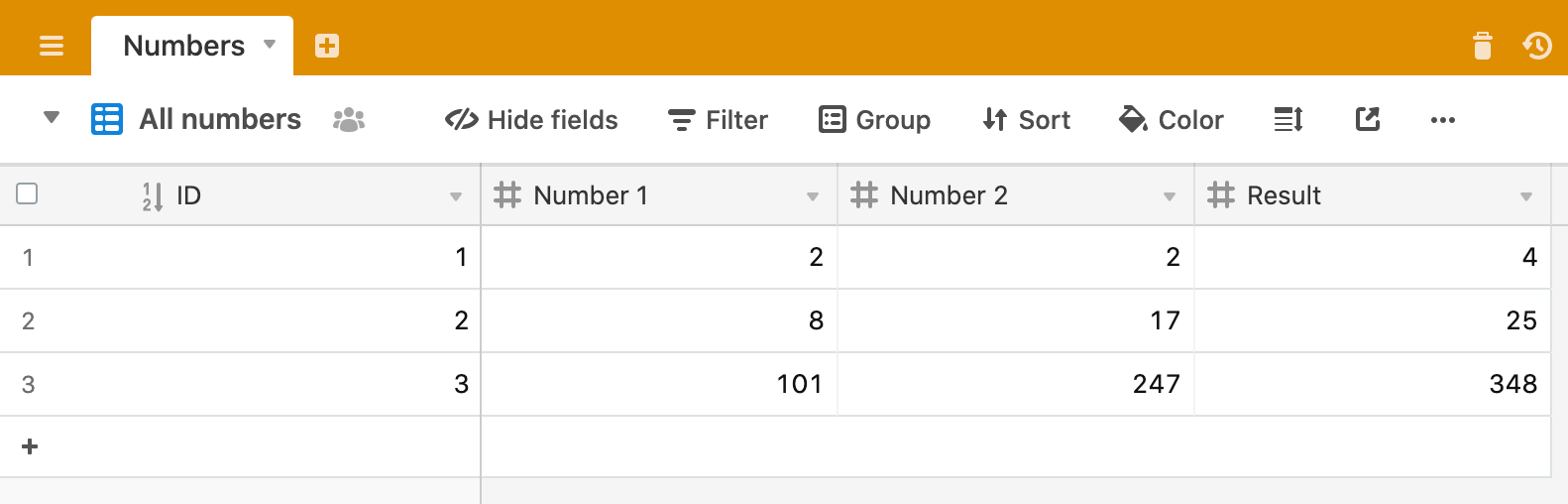### AirscriptLearn to use Airtable scripts

In this script we’re going to take two numbers, from two different fields in our table, add the together and write the result to the 3rd field.Of course, you wouldn’t normally do this in your Airtable base as you could simply create a formula field that added two other fields together. But this blog is about scripting, so we’ll do it anyway 😀.

Open your scripting block and clear any code that’s there. Let’s start in the normal way by defining the table we’re going to use and query the records from this table.

``````
let table = base.getTable('Numbers');
let query = await table.selectRecordsAsync();

``````

We’re going to iterate through the records, add them together and write them to the “Result” field, but let’s start simply by iterating through the records and printing out the values.

``````
let table = base.getTable('Numbers');
let query = await table.selectRecordsAsync();

for (let record of query.records) {
let number1 = record.getCellValue('Number 1');
let number2 = record.getCellValue('Number 2');
console.log(number1, number2);
}

``````

Here, we’re defining two variables, `number1` and `number2` and setting their values to be the value in field “Number 1” and field “Number 2” respectively. Then we are simply logging the two variables for each record to the output window. Run the script and you will see something like this:Now, instead of just logging the two field values for each record, let’s define a result variable and set the value of this to be `number1` and `number2` added together. We’ll also log the new `result` variable for each record so that we can see that it is working as we expect it to:

``````
let table = base.getTable('Numbers');
let query = await table.selectRecordsAsync();

for (let record of query.records) {
let number1 = record.getCellValue('Number 1');
let number2 = record.getCellValue('Number 2');
let result = number1 + number2;
console.log(number1, number2, result);
}

``````

And the output should be something like this:Perfect! This gives us a quick visual check that it is working and we haven’t made any mistakes. Yes, 2+2 does, in fact, equal 4.

So, our final step is to take the result variable for each record we iterate through and write it to the “Result” field. Here we will use another Airtable script method - `table.updateRecordAsync`.

The `updateRecordAsync` method needs two parameters or arguments to be passed to it. It needs to know which record it will update and what data to update it with. Let’s look at what this looks like for our adding numbers script, then we’ll break it down to explain the different parts.

``````
let table = base.getTable('Numbers');
let query = await table.selectRecordsAsync();

for (let record of query.records) {
let number1 = record.getCellValue('Number 1');
let number2 = record.getCellValue('Number 2');
let result = number1 + number2;
await table.updateRecordAsync(record, {
"Result": result
});
}

``````

In the above code, you can see the two parameters passed in the `updateRecordAsync` method. The first parameter is `record` which identifies the record we want to update. Remember that record is our iteration variable, so on each iteration, `record` represents a different record from our table.

The second parameter is this:

``````
{
"Result": result
}
``````

What’s going on here?

When we want to create or update records in Airtable, we have to pass this data as an “object” (identified by a set of key/value pairs enclosed in curly braces). In our data object, we’re noting that `Result`, the name of our field in the table, will have the value represented by `result`, which, like `record` above, has a new calculated value for each iteration of the loop.

Run the script and watch as the “Result” field is updated with the sum of the other two numbers.We can do two more things to make the script more professional. The first is a matter of user experience. When the script runs, it whirs away, but you don’t see much happening until it is finished. It is nice (and common practice) to give the user some indication that good stuff is happening, or, if there are errors, to alert the user to these.

We can add some `output.text` on each iteration to indicate to the user that the record has been updated:

``````
let table = base.getTable('Numbers');
let query = await table.selectRecordsAsync();

for (let record of query.records) {
let number1 = record.getCellValue('Number 1');
let number2 = record.getCellValue('Number 2');
let result = number1 + number2;
await table.updateRecordAsync(record, {
"Result": result
});
output.text(`Record \${record.name} has been updated!`)
}

``````

Note that the output text here uses Template Strings to output a combination of regular text and a variable, in this case, our `record.name`. Run the script again to see the result.

Our final enhancement is another piece of good practice and will result in the script only calculating the sum of our two numbers if it hasn’t already been calculated (on a prior execution of the script, for example). We still want to iterate through all of the records, so that we can figure out which records need calculating and which don’t, but we’ll use an `if` statement to find records that don’t have a “Result” value and will only run the addition code for these records.

`if` statements take the form:

``````
if (condition) {
// code to be executed if the condition is true
}

``````

As we start each iteration of the loop, we’ll use the `if` statement to check if something is true, in this case that the “Result” field is currently empty.

Our updated code looks like this:

``````
let table = base.getTable('Numbers');
let query = await table.selectRecordsAsync();

for (let record of query.records) {
if (!record.getCellValue('Result')) {
let number1 = record.getCellValue('Number 1');
let number2 = record.getCellValue('Number 2');
let result = number1 + number2;
await table.updateRecordAsync(record, {
"Result": result
});
output.text(`Record \${record.name} has been updated!`)
}
}

``````

Our code is now saying:

“For each record of query.records, if “Result” field of this record is empty, then add the numbers and update the “Result” field”

The exclamation mark (“!”) is the logical operator “not”, so we’re saying:

`if (not Result) then...`

Delete the result from one of the rows in your table and run the script again. You should now see that only one of the records is updated.

## Recap

• Getting records from your table and looping through them
• Defining a variable that is the sum of two other numbers
• Updating a record with `table.updateRecordAsync`
• Adding some output to indicate progress to the user
• Only acting upon those records that need to be acted upon